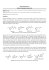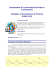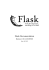# Chemical Kinetics AP Problems Quiz Numbers 1 to6

## Transcription

Chemical Kinetics AP Problems Quiz Numbers 1 to6
```Name #1:_____________________________ Name #2:_____________________________
Chemical Kinetics AP Problems Problem #1
1. In an experiment, all the air in a rigid 2.0 L flask is pumped out. Then some liquid ethanol is injected into the
sealed flask, which is held at 35 oC. The amount of liquid ethanol initially decreases, but after five minutes the
amount of liquid ethanol in the flask remains constant. Ethanol has a boiling point of 78.5 oC and an equilibrium
vapor pressure of 100 torr at 35 oC.
a. When the amount of liquid ethanol in the flask is constant, is the pressure in the flask greater than, less
than, or equal to 100 torr at 35 oC? Justify your answer.
b. The flask is then heated to 45 oC, and the pressure in the flask increases. In terms of kinetic molecular
theory, provide TWO reasons that the pressure in the flask is greater at 45oC than at 35 oC.
In a second experiment, which is performed at a much higher temperature, a sample of ethanol gas and a copper
catalyst are placed in a rigid, empty 1.0 L flask. The temperature of the flask is held constant, and the initial
concentration of the ethanol gas is 0.0100 M. The ethanol begins to decompose according to the chemical reaction
represented below.
CH3CH2OH(g) Cu⇢ CH3CHO(g) + H2(g)
The concentration of ethanol gas over time is used to create the three graphs below.
c.
Given that the reaction order is zero, one, or two, use the information in the graphs to respond to the
following.
i. Determine the order of the reaction with respect to ethanol. Justify your answer.
ii. Write the rate law for the reaction.
iii. Determine the rate constant for the reaction, including units.
d. The pressure in the flask at the beginning of the experiment is 0.40 atm. If the ethanol completely
decomposes, what is the final pressure in the flask?
Name #1:_____________________________ Name #2:_____________________________
Chemical Kinetics AP Problems Problem #2
8 H+(aq) + 4 Cl-(aq) + MnO4- (aq) ⇢ 2 Cl2(g) + Mn 3+(aq) + 4 H2O(l)
2. Cl2(g) can be generated in the laboratory by reacting potassium permanganate with an acidified solution of
sodium chloride. The net-ionic equation for the reaction is given above.
a. A 25.00 mL sample of 0.250 M NaCl reacts completely with excess KMnO4(aq). The Cl2 (g) produced is
dried and stored in a sealed container. At 22°C the pressure of the Cl2(g) in the container is 0.950 atm.
i. Calculate the number of moles of Cl-(aq) present before any reaction occurs.
ii. Calculate the volume, in L, of the Cl2 (g) in the sealed container.
An initial-rate study was performed on the reaction system. Data for the experiment are given in the table below.
Trial
[Cl-]
[MnO4-]
[H+]
Rate of Disappearance of MnO4- in M s-1
1
2
3
0.0104
0.0312
0.0312
0.00400
0.00400
0.00200
3.00
3.00
3.00
2.25 x 10-8
2.03 x 10-7
1.02 x 10-7
b. Using the information in the table, determine the order of the reaction with respect to each of the
i. Cl-(aq)
ii. MnO4- (aq)
c. The reaction is known to be third order with respect to H+. Using this information and your answers to
part (b) above, complete both of the following:
i. Write the rate law for the reaction.
ii. Calculate the value of the rate constant, k, for the reaction, including appropriate units.
d. Is it likely that the reaction occurs in a single elementary step? Justify your answer.
Name #1:_____________________________ Name #2:_____________________________
Chemical Kinetics AP Problems Problem #3
H2(g) + Cl2 (g) ⇢ 2 HCl(g)
3. The table below gives data for a reaction rate study of the reaction represented above.
Experiment
Initial [H2 ]
Initial [Cl2 ]
Initial Rate of Formation of
mol L
mol L
HCl mol L s
1
0.00100
0.00500
1.82 x 1012
2
0.00200
0.00500
3.64 x 1012
3
0.00200
0.00250
1.82 x 1012
a. Determine the order of the reaction with respect to H2 and justify your answer.
b. Determine the order of the reaction with respect to Cl2 and justify your answer.
c. Write the overall rate law for the reaction.
d. Write the units of the rate constant.
e. Predict the initial rate of the reaction if the initial concentration of H2 is 0.00300 mol L and the initial
concentration of Cl2 is 0.00500 mol L .
The gas-phase decomposition of nitrous oxide has the following two-step mechanism.
Step 1: N2O ⇢ N2 + O
f.
Step 2: O + N2O ⇢ N2 + O2
Write the balanced equation for the overall reaction.
g. Is the oxygen atom, O, a catalyst for the reaction or is it an intermediate? Explain.
h. Identify the slower step in the mechanism if the rate law for the reaction was determined to be
Name #1:_____________________________ Name #2:_____________________________
Chemical Kinetics AP Problems Problem #4
4. An environmental concern is the depletion of O3 in Earth’s upper atmosphere, where O3 is in normal equilibrium
with O2 and O. A proposed mechanism for the depletion of O3 in the upper atmosphere is shown below
Step I
O3 + Cl ⇢ O2 + ClO
Step II
ClO + O ⇢ Cl + O2
a. Write a balanced equation for the overall reaction represented in Step I and Step II above.
b. Clearly identify the catalyst in the mechanism above. Justify your answer.
c. Clearly identify the intermediate in the mechanism above. Justify your answer.
d. If the rate law for the overall reaction is found to be rate = k [O3] [Cl], determine the following.
i. The overall order of the reaction.
ii. Appropriate units for the rate constant, k.
iii. The rate-determining step of the reaction, along with justification for your answer
Name #1:_____________________________ Name #2:_____________________________
Chemical Kinetics AP Problems Problem #5
X(g)
kf
kr
A (g)
B(g)
H
15 kilocalories
5. The forward reaction shown above is slow at room temperature but becomes rapid when a catalyst is added.
a. Draw a diagram of potential energy vs reaction coordinate for the uncatalyzed reaction below. On this
diagram below label the following:
i. the axes
ii. the energies of the reactants and the products
iii. the energy of the activated complex
iv. all significant energy differences
b. On the same diagram indicate the change or changes that result from the addition of the catalyst. Explain
the role of the catalyst in changing the rate of the reaction.
c. Changing the temperature and no other conditions changes the rates of most chemical reactions. Two
factors are commonly cited as accounting for the increased rate of chemical reaction as the temperature is
increased. State briefly and discuss the two factors. Which of the two is more important?
Name #1:_____________________________ Name #2:_____________________________
Chemical Kinetics AP Problems Problem #6
2A+2B
C+D
6. The following data about the reaction above were obtained from three experiments:
Initial Rate of Formation of C
(mole.liter-1min-1)
Experiment
[A]
[B]
1
0.60 0.15
6.3 10-3
2
0.20 0.60
2.8 10-3
3
0.20 0.15
7.0 10-4
d. What is the order of the reaction with respects to each reactant? Support your answer
i. A
ii. B
e. Write a rate law for the reaction.
f.
What is the numerical value of the rate constant k? What are its units?
g. Propose a reaction mechanism for this reaction.
```

### 1. Define spontaneous generation. The idea that a mysterious life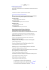### Ultimate Gas Laws Worksheet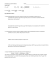### BluZy®-P XL UNLEASH THE FULL VALUE OF YOUR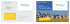### Untitled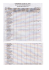### Ch 223 Practice Exam #1 PART I: Multiple Choice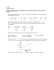### 251. The Fleaker - UC DRC Home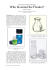### Kinetics Worksheet - Mro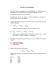### Louise Irvine, the renowned Royal Doulton expert and author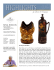### Document 6529186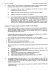### a new era begins - European Biofuels Technology Platform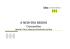### What is the charge of Zn in the compounds ZnCl Zn(OH)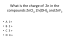### CELLOSIC ETHANOL IGPC Ethanol Inc.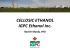### Handout 7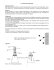### Wellbutrin/Zyban synthesis